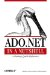# 6.2 Working with Tables in the DataSet

 Tables belonging to the DataSet are stored as DataTable objects in a DataTableCollection object and accessed through the Tables property of the DataSet . This section examines some methods and properties of the DataTableCollection . Tables are added to the DataSet using the Add( ) method of the DataTableCollection . The Add( ) method takes an optional table name argument. If this argument isn't supplied, the tables are automatically named Table , Table1 , and so on. The following example adds a table to a DataSet : ` DataSet ds = new DataSet("MyDataSet"); DataTable dt = new DataTable("MyTable"); // ... code to define the schema for the newly constructed DataTable ds.Tables.Add(dt); ` The AddRange( ) method allows more than one table to be added to the DataSet in the same statement. The method takes an array of DataTable objects as the argument, as the following example shows: ` // create two new tables DataTable dt1 = new DataTable(); DataTable dt2 = new DataTable(); // use the AddRange() method to add them to the DataSet. ds.Tables.AddRange(new DataTable[] {dt1, dt2}); ` A DataTable can also be created automatically in a DataSet when the Fill( ) or FillSchema( ) method of the DataAdapter is called. A new table is created and filled with the data or schema, respectively, from the data source, as illustrated in the following code: ` // connection and select command strings String connString = "Data Source=(local);Integrated security=SSPI;" + "Initial Catalog=Northwind;"; String selectSql = "SELECT * FROM Orders"; // create a new DataSet to receive the data DataSet ds = new DataSet(); SqlDataAdapter da = new sqlDataAdapter(selectSql, connString); // an empty table named OrdersSchema will be created in the DataSet da.FillSchema(ds, SchemaType.Mapped, "OrdersSchema"); // a table named Orders will be created in the DataSet // filled with data as specified by the SQL statement da.Fill(ds, "Orders"); ` The DataAdapter class and the Fill( ) and FillSchema( ) methods are discussed in detail in Chapter 14. Existing tables within the DataSet can be accessed by an indexer, which usually is passed the table name or the position of the table within the DataTableCollection as an argument as shown in the following examples: ` // using the table name DataTable dt = ds.Tables["MyTable"]; // using the table ordinal DataTable dt = ds.Tables; ` The Count property returns the number of tables within the DataSet : ` Int32 tableCount = ds.Tables.Count; ` The Contains( ) method determines whether a table with a specified table name exists within a DataSet : ` // Boolean tableExists = ds.Tables.Contains("MyTable"); ` The IndexOf( ) method returns the index of the table within the collection using either a reference to the table object or the name of a table. The following example demonstrates both techniques: ` // get the index using the name of the table Int32 tableIndex = ds.Tables.IndexOf("MyTable"); // get the index using a reference to a table DataTable dt = ds.Tables.Add("MyTable") // ... build the table and do some work // get the index of the table based on the table reference Int32 tableIndex = ds.Tables.IndexOf(dt); ` The Remove( ) , RemoveAt(), and Clear( ) methods remove tables from the DataSet . The Remove( ) method takes an argument that specifies either a table name or a reference to the table to be removed, as shown in the following example: ` DataTable dt = ds.Tables.Add("MyTable"); // remove by table reference ds.Remove(dt); // remove using the table name ds.Remove("MyTable"); ` The RemoveAt( ) method removes the table at the specified index from the DataTableCollection object, as shown in the following example: ` // removes the first table from the tables collection in the DataSet ds.RemoveAt; ` The Clear( ) method removes all tables from the DataSet , as shown here: ` ds.Tables.Clear(); `